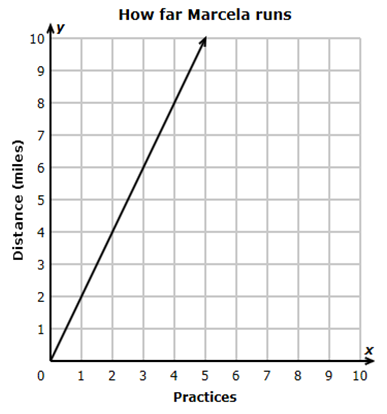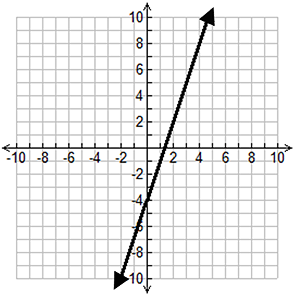Constant of Proportionality

My Definition

Key Characteristics

The following are true of the constant of proportionality:

• It is represented symbolically by the variable k.

• It describes the constant ratio of two proportional quantities, usually represented with x and y such as k =
yx
.

• It is also known as constant of variation or unit rate.

• It describes the constant rate of change between two quantities in a proportional relationship.

• It represents the slope of the line of a graphed proportional relationship where x represents the independent variable and y represents the dependent variable.

Example

Video Transcript (PDF)

Use the link below to further study examples of Constant of Proportionality.

Constant of Proportionality ActivityNon-example

In the equation y = 3x - 4, three is the slope in a non-proportional situation.TEKS: 7(4)(C), 8(5)(E)